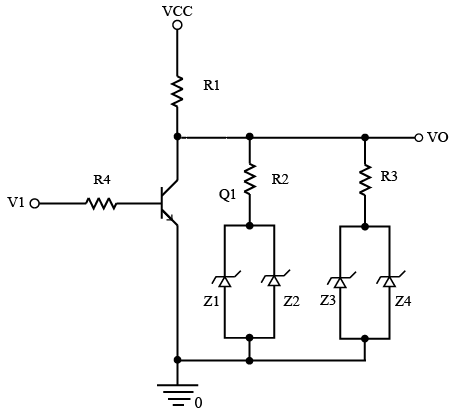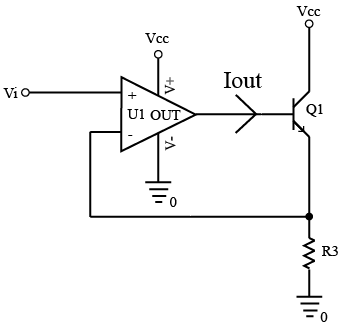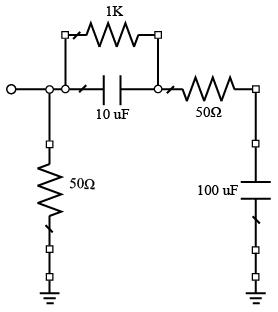# ISRO Scientist or Engineer Electronics 2010

Instructions

For the following questions answer them individually

Question 51

# A microprocessor has a cache memory with access time of 2 ns and a main memory with access time of 10 ns. If the cache missratio is 0.6, what is the average memory access time?Question 52

# Consider the following assembly code for a hypothetical RISC processor with a 4-stage pipeline (Instruction Fetch, Decode/Register Read, Execute and Write).add $$r_{1},r_{2},r_{3} \parallel r_{1} = r_{2} + r_{3}$$ sub $$r_{4},r_{1},r_{3} \parallel r_{4} = r_{1} - r_{3}$$ mul $$r_{5},r_{2},r_{3} \parallel r_{5} = r_{2} \star r_{3}$$ Identify the possible pipeline hazard and the affected instruction.Question 53

# A zero memory source emits six messages with probabilities 0.3, 0.25, 0.15, 0.12, 0.1 and 0.08. If binary Huffmancodingis used, what will be the average code length?Question 54

# In the circuit given below, assume that $$V_{cc}= 15V; Z_{1}, Z_{2}, Z_{3} \& Z_{4}$$ are identical zener diodes with breakdownvoltage of 5V; $$R_{1} = R_4 = 5kΩ, R_{2} = R_{3} = 10kΩ$$. Find $$V_{o}$$ when $$Q_{1}$$ is OFF.Question 55

# Assume that $$V_{i}= 2V; R_{3} = 10Ω; \beta$$ of $$Q_{1} = 50; V_{cc} = 15V$$. Find $$I_{out}$$.Question 56

# A sample and hold amplifier is connected to an ADC. The acquisition time of the sample and hold amplifier is 10 usec and the conversion time of the ADCis 15 psec. What is the highest possible data rate?Question 57

# In an inverting OP-AMP,the input bias currentis -1$$\mu$$A, and the input and the feedback resistances are both 1MQ. What will be the output voltage for an input voltage of 2.5V?Question 58

# Which of the following devices can be used for generating a PWM signal?Question 59

# A microwavereceiving antenna on a satellite is pointing towards the earth. The antenna beamwidth is 50 degrees. The earth subtends 5 degreesat the satellite. What will be the noise temperature of the antenna?Question 60

# Estimate the magnitude and phase of the element $$S_{11}$$ of the S-matrix of the circuit shown below. Assume an operating frequency of 25 MHz.OR